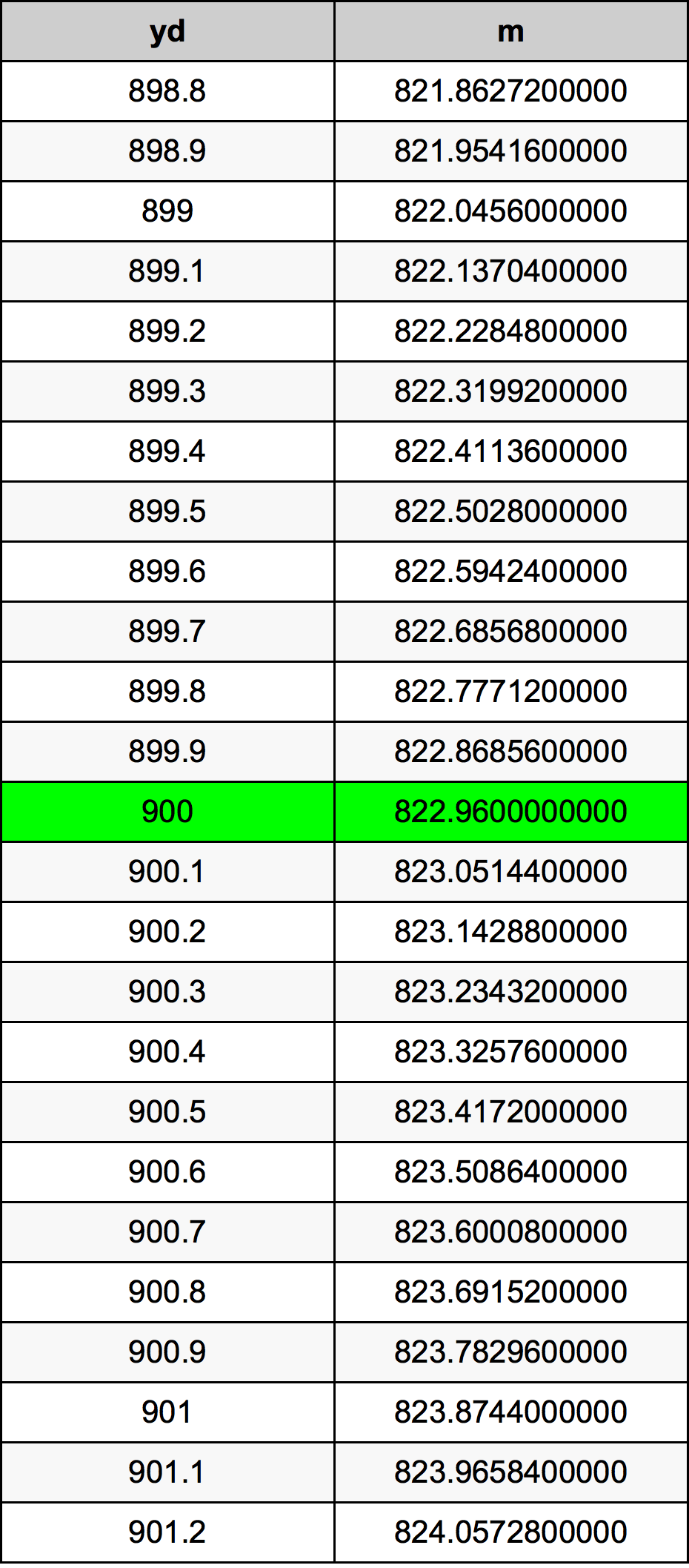Yards To Meters

# 900 yd to m900 Yards to Meters

yd
=
m

## How to convert 900 yards to meters?

 900 yd * 0.9144 m = 822.96 m 1 yd
A common question is How many yard in 900 meter? And the answer is 984.251968504 yd in 900 m. Likewise the question how many meter in 900 yard has the answer of 822.96 m in 900 yd.

## How much are 900 yards in meters?

900 yards equal 822.96 meters (900yd = 822.96m). Converting 900 yd to m is easy. Simply use our calculator above, or apply the formula to change the length 900 yd to m.

## Convert 900 yd to common lengths

UnitLength
Nanometer8.2296e+11 nm
Micrometer822960000.0 µm
Millimeter822960.0 mm
Centimeter82296.0 cm
Inch32400.0 in
Foot2700.0 ft
Yard900.0 yd
Meter822.96 m
Kilometer0.82296 km
Mile0.5113636364 mi
Nautical mile0.444362851 nmi

## What is 900 yards in m?

To convert 900 yd to m multiply the length in yards by 0.9144. The 900 yd in m formula is [m] = 900 * 0.9144. Thus, for 900 yards in meter we get 822.96 m.

## 900 Yard Conversion Table## Alternative spelling

900 yd to m, 900 yd in m, 900 Yard to Meters, 900 Yard in Meters, 900 Yard to Meter, 900 Yard in Meter, 900 yd to Meters, 900 yd in Meters, 900 Yards to Meters, 900 Yards in Meters, 900 yd to Meter, 900 yd in Meter, 900 Yards to Meter, 900 Yards in Meter